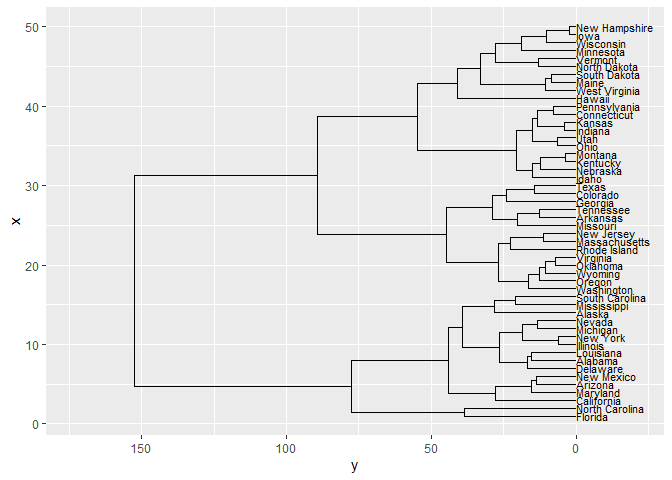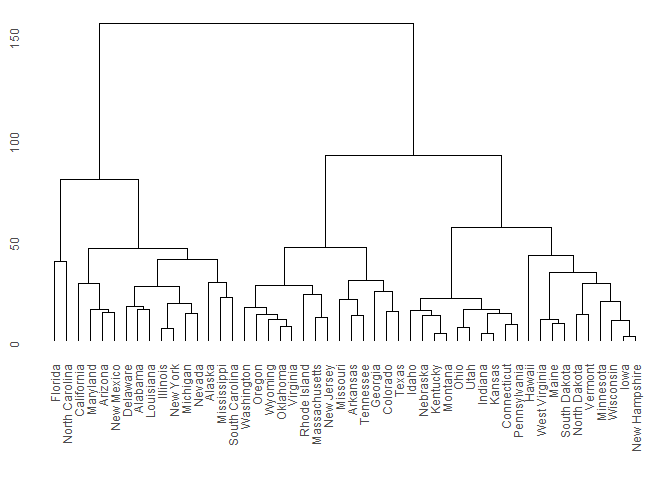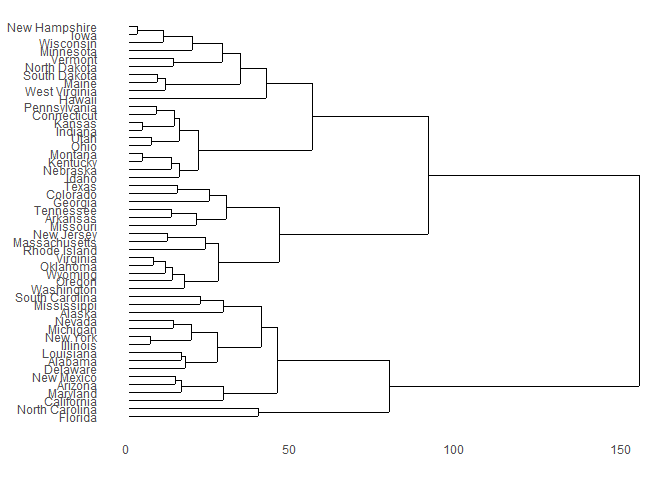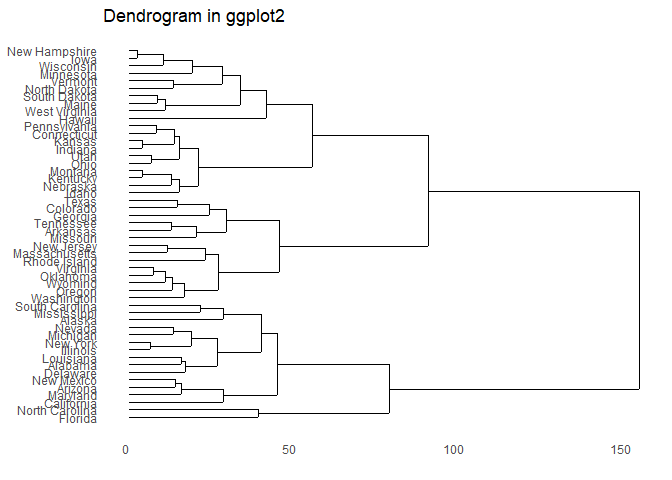Provides functions for creating dendrograms and tree plots using ggplot2.

The ggdendro package offers a generic function to extract data and text from the various clustering models:

• dendro_data() extracts cluster information from the model object, e.g. cluster allocation, line segment data or label data.

The dendro_data object has methods for the following classes:

• tree
• hclust
• dendrogram
• rpart

These methods create an object of class dendro, which is essentially a list of data frames. To extract the relevant data frames from the list, use the three accessor functions:

• segment() for the line segment data
• label() for the text for each end segment
• leaf_label() for the leaf labels of a tree diagram

The results of these functions can then be passed to ggplot() for plotting.

## Example

library(ggplot2)
library(ggdendro)
hc <- hclust(dist(USArrests), "ave")
hcdata <- dendro_data(hc, type = "rectangle")
ggplot() +
geom_segment(data = segment(hcdata),
aes(x = x, y = y, xend = xend, yend = yend)
) +
geom_text(data = label(hcdata),
aes(x = x, y = y, label = label, hjust = 0),
size = 3
) +
coord_flip() +
scale_y_reverse(expand = c(0.2, 0))### demonstrate plotting directly from object class hclust
ggdendrogram(hc)ggdendrogram(hc, rotate = TRUE)### demonstrate converting hclust to dendro using dendro_data first
hcdata <- dendro_data(hc)
ggdendrogram(hcdata, rotate = TRUE) +
labs(title = "Dendrogram in ggplot2")